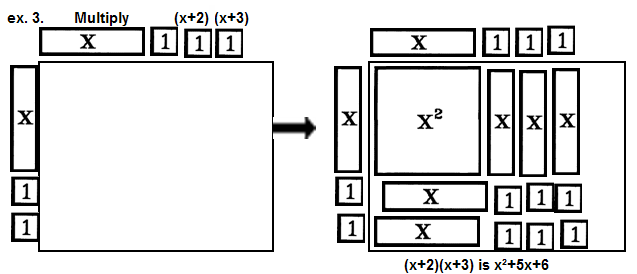The Visual / Auditory / Symbolic / Kinesthetic Approach to Algebra

 Distributive Property of Multiplication over Addition

In algebra, the distributive property is required for the multiplication of even a monomial by a binomial. In arithmetic, the distributive property is used but not required.

To set the stage for OPERATIONS (addition, multiplication, division, factoring, etc.) work with REPESENTATION (represent, write, show) is very important. If you have not worked with representing and writing expressions, please do so before continuing with multiplication.

Example 1, below, illustrates how the distributive property develops naturally from work with representing and writing algebraic expressions. Consider how representing and writing an expression for "double the sum of a number and three" evolves into "multiply 2 times x+3."

 Algebraic Multiplication

MULTIPLICATION
 1st: Represent the first number, the minuend.     Use a blank rectangular sheet of paper to separate the storage region from the display area and to create a border around the answer. 2nd: Represent one factor (usually the fist represented in descending order) vertically in the storage on the left edge of the display area. The factor acts as a measure of the length of the side. 3rd: Represent the other factor (in descending order) horizontally in the storage on the top edge of the display area. The factor acts as a measure of the length of the side. 4th: Multiply a top tile by a side tile filling in the corresponding region of the display area with the required tile. These tiles represent the product and the area of the region. 5th: The product is produced in the display area.

Multiply.Example 3 is even more complicated. It illustrates that distributing a binomial over another binomial is what is usually called multiplying two binomials.Example 3 edited in red at the right, displays the traditional "first, outer, inner, last" format for binomial multiplication called FOIL.This author teaches FOIL, prefers FLOI for its use in multiplication of complex numbers, but, emphasizes most that as long as 4 multiplications are completed, it doesn’t matter what order the multiplications are performed.

Example 4 illustrates that removing zeros might be required.Example 5 "looks funny" and is NOT USUALLY DISCUSSED MANIPULATIVELY. It emphasizes the fact that the displayed product is really the sum of the areas no matter what shape that area takes.Example 6 can't be discussed manipulatively with area TILES. The product involves a cube, a volume. The xł tile or token represents a 3 dimensional idea in a 2 dimensional way. A volume TOKEN is required.The symbol on the cube says: This is a cube with a volume of xł. Its base has an area of x˛ and its height is x.

Using the cube token, products not normally considered with manipulatives may be examined. Read "Special Products".www.termtiles.com, Unit 17   © 2008, A. Azzolino